##### Basic Math & Pre-Algebra For Dummies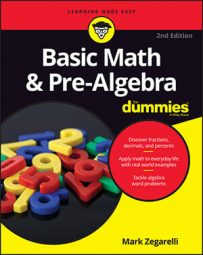Scientific notation is a standard way of writing very large and very small numbers so that they're easier to both compare and use in computations. To write in scientific notation, follow the form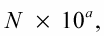where N is a number between 1 and 10, but not 10 itself, and a is an integer (positive or negative number).

You move the decimal point of a number until the new form is a number from 1 up to 10 (N), and then record the exponent (a) as the number of places the decimal point was moved. Whether the power of 10 is positive or negative depends on whether you move the decimal to the right or to the left. Moving the decimal to the right makes the exponent negative; moving it to the left gives you a positive exponent. To see an exponent that's positive, write 312,000,000,000 in scientific notation:

1. Move the decimal place to the left to create a new number from 1 up to 10.

Where's the decimal point in 312,000,000,000? Because it's a whole number, the decimal point is understood to be at the end of the number: 312,000,000,000.

So, N = 3.12.

2. Determine the exponent, which is the number of times you moved the decimal.

In this example, you moved the decimal 11 times; also, because you moved the decimal to the left, the exponent is positive. Therefore, a = 11, and so you get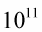3. Put the number in the correct form for scientific notation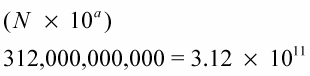To see an exponent that's negative, write .00000031 in scientific notation.
1. Move the decimal place to the right to create a new number from 1 up to 10.

So, N = 3.1.

2. Determine the exponent, which is the number of times you moved the decimal.

In this example, you moved the decimal 7 times; also, because you moved the decimal to the right, the exponent is negative. Therefore, a = –7, and so you get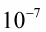3. Put the number in the correct form for scientific notation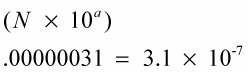When you get used to writing numbers in scientific notation, you can do it all in one step. Here are a few examples: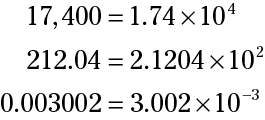## Order of magnitude

Why does scientific notation always use a decimal between 1 and 10? The answer has to do with order of magnitude, which is a simple way to keep track of roughly how large a number is so you can compare numbers more easily. The order of magnitude of a number is its exponent in scientific notation. For example,

703 = 7.03 x 102 — order of magnitude is 2 600,000 = 6 x 105 — order of magnitude is 5 0.00095 = 9.5 x 10–4 — order of magnitude is –4

Every number between 10 and 100 has an order of magnitude of 1. Every number between 100 and 1,000 has an order of magnitude of 2.